##### Algebra II For Dummies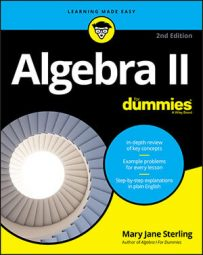When solving rational inequalities, you use the same steps as for any quadratic inequality. By adding the sign-line method, you can also learn whether the different factors in each interval are positive or negative.

Begin with these steps:

1. Move all the terms to one side of the inequality sign.

2. Factor, if possible.

3. Determine all zeros (roots, or solutions).

Zeros are the values of the variable that make each factored expression equal to zero.

4. Put the zeros in order on a number line.

5. Create a sign line to show where the expression in the inequality is positive or negative.

A sign line shows the signs of the different factors in each interval. If the expression is factored, show the signs of the individual factors.

6. Determine the solution, writing it in inequality notation or interval notation.

The signs of the results of multiplication and division use the same rules, so to determine your answer, you can treat the numerator and denominator the same way you treat two different factors in multiplication. Here's an example: solve the rational inequality,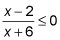You can use these steps to find the solution for this rational inequality:

1. Every term in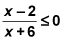is to the left of the inequality sign.

2. Neither the numerator nor the denominator factors any further.

3. The two zeros are x = 2 and x = –6.

4. Draw the two numbers on a number line.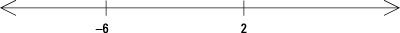5. Create a sign line for the two zeros; you can see here that the numerator is positive when x is greater than 2, and the denominator is positive when x is greater than –6.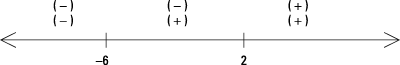6. When determining the solution, keep in mind that the inequality calls for something less than or equal to zero.

The fraction is a negative number when you choose an x between –6 and 2. You get a negative numerator and a positive denominator, which gives a negative result. Another solution to the original inequality is the number 2. Letting x = 2, you get a numerator equal to 0, which you want because the inequality is less than or equal to zero. You can't let the denominator be zero, though. Having a zero in the denominator isn't allowed because no such number exists. So, the solution of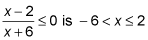In interval notation, you write the solution as (–6, 2].